Mesh method for solving boundary value problem of the mixed type using Mathematica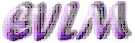Anotation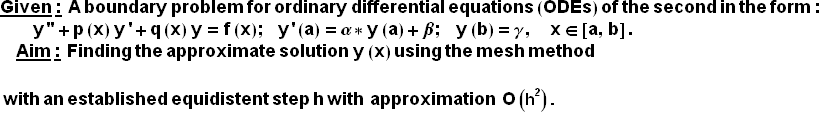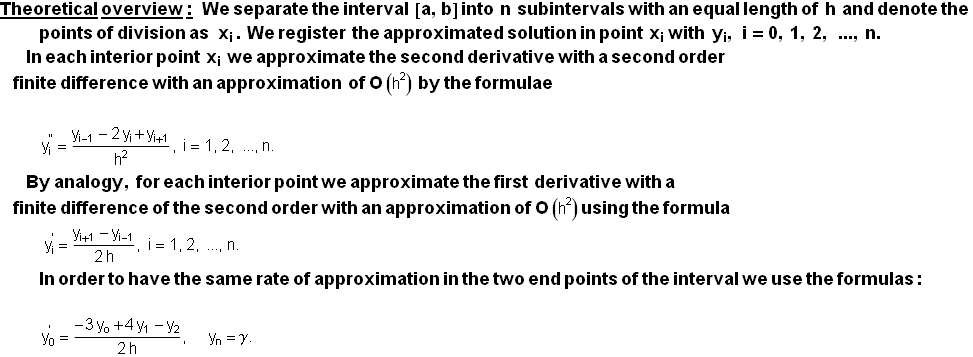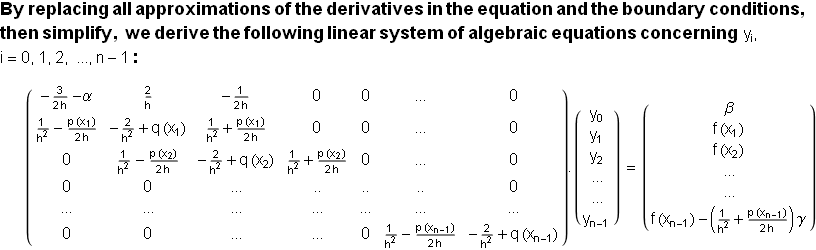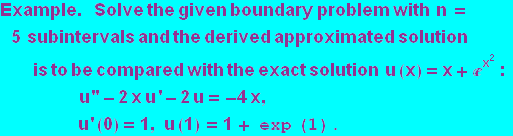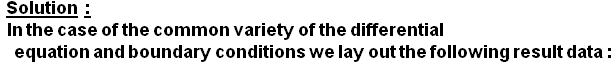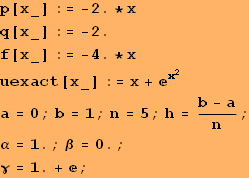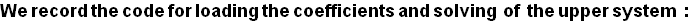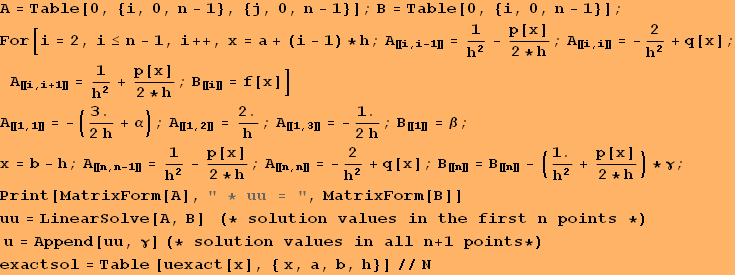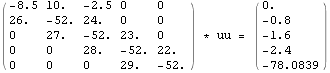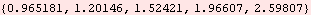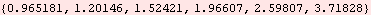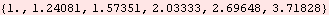Graphic representation of the solution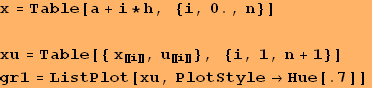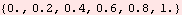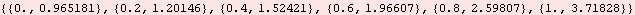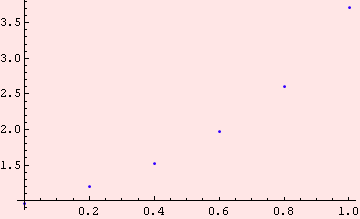Comparison with the exact solution and real errors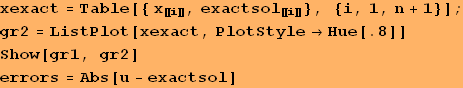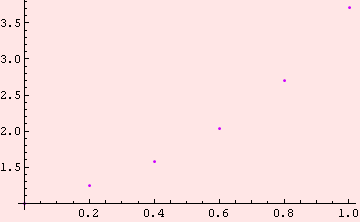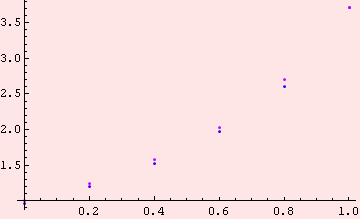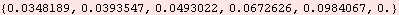Conclusion
Since in this example h = 0.2 and the approximation is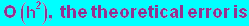of the order approximate to 0.04.
The real error of the next upper line is 0.1. That is explained with the upper boundary of the derivative of the third order of the exact solution (see more detailed theory).
Recommendation. In order to supply the required error for an arbitrary problem we may reduce the step h until two consecutive approximations in their respective  points match the needed accuracy.

 Created by Wolfram Mathematica 6.0  (21 September 2008)